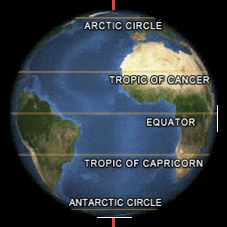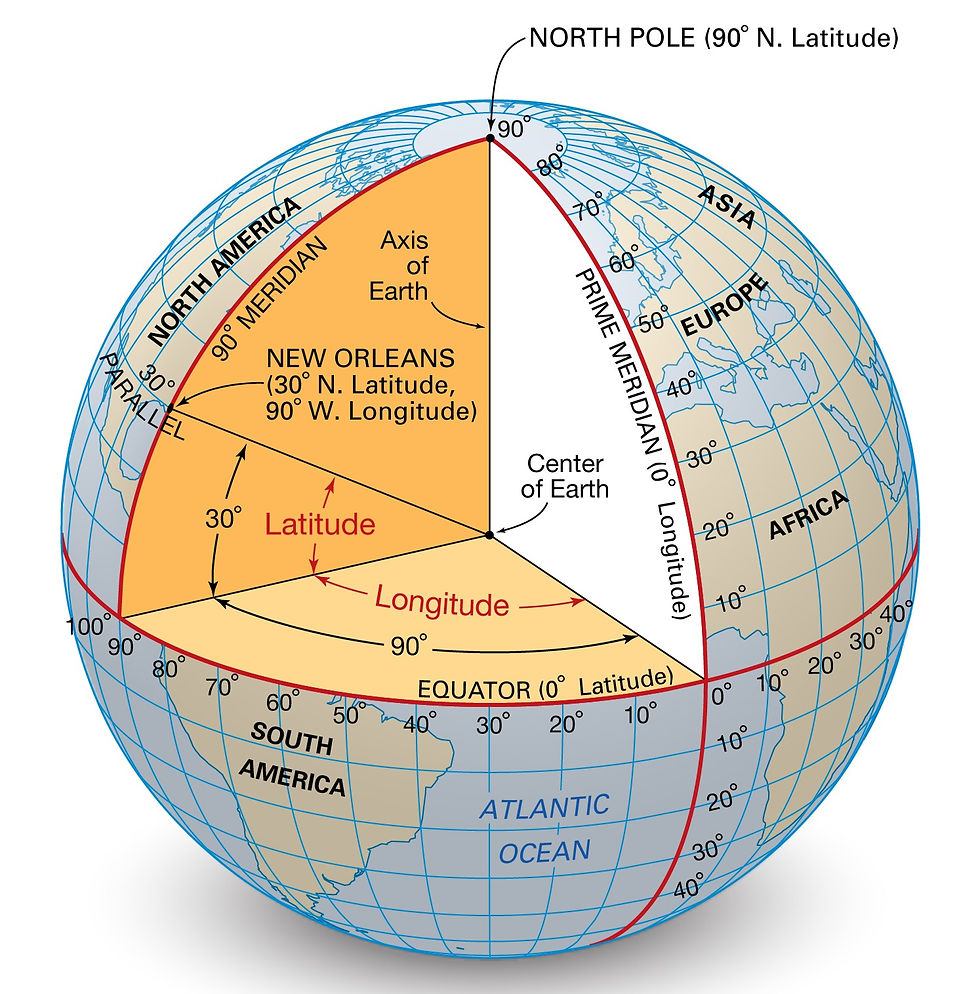top of pageSearch

# Do you know how Locations are defined on Earth? UPSC Physical geography #Latitudes #Longitudes

Updated: Jun 10, 2021

You might have opened google maps some time and wondered how those coordinates are defined. Let's address your curiosity. The basics come from the concept of latitudes and longitudes.

Let's Start :

1. Equator: This is a line drawn on the circumference of the earth which is at equal distance from both the poles ie. the north pole and the south pole and the center of the earth and divides the earth into two equal halves. For geographical reference, the equator has a latitude of 0 degrees.Now we draw circles around the Earth parallel to the equator running east to west on both sides of the equator. These circles are called parallels of Latitude and are expressed through angles. So all the places that lie on a parallel have the same latitude. In total, we draw 180 such circles or rings around the earth. So we have in total 180 latitudes.

2. Latitude: It is the measurement of distance north or south of the Equator. A geographic coordinate that specifies the north-south position of a point on the Earth's surface. Latitude is an angle that ranges from 0° at the Equator to 90° (North or South) at the poles.

Went above the head? Wait let me explain!

Refer to the image below. Imagine the center point of the Earth which would be at the mid of the inner core. Now from that center try to draw a line to a point on the equator. Now at what angle would this line be from the horizontal considering a 2D plane? It would definitely be zero. Why? because the center of the earth and the equator line in the same plane, so the line joining the center to any point on the equator would be in the same plane and at zero degrees from horizontal. Now, let's move towards the north, remember we drew several circles parallel to the equator and these would define our latitude.Now we move towards the north and encounter a circle. Let us join the center of the earth to any point on this circle. What angle would this line make with the horizontal or equator? let's say it makes 46 degrees so now we have defined our latitude which is 46 degrees to the north of the equator .since we went north this is expressed as 46 degrees N. In a similar case, we can go south of the equator and encounter a similar circle making 46 degrees with the equator and that would be named 46 Degrees S.

Now we know that maximum we can go to poles and the line from center to the pole would make 90 degrees from the equator. We would call that 90 degrees N. So our north pole has a latitude of 90 degrees N and the south pole has a latitude of 90 degrees S. We draw circles at 1 degree each from the equator due to which we have 90 circles from the equator to the north pole and 90 circles from the equator to the south pole making it total 180 such latitudes.

Some important latitudes: The five major parallels of latitudes from north to south are called: Arctic Circle, Tropic of Cancer, Equator, Tropic of Capricorn, and the Antarctic Circle.

Equator: The equator crosses 78.7% water and 21.3% land and is about 24,901 miles (40,075 km) long.

Tropic of Cancer: Its latitude is 23° 26′ (23.43°) N. It marks the location where the sun reaches the zenith at this latitude in the northern hemisphere. This means the sun never goes overhead beyond this latitude in the northern hemisphere. Sun is overhead over the Tropic of cancer on either June 20 or 21 of each year.

Tropic of Capricorn: It's like the mirror image of the tropic of cancer in the southern hemisphere. Its latitude is 23° 26′ (23.43°) S. It marks the location where the sun reaches the zenith at this latitude in the Southern hemisphere. This means the sun never goes overhead beyond this latitude in the southern hemisphere. Sun is overhead over the Tropic of Capricorn on either December 21 or 22 of each year.

So Tropics mark the limit to which the sun can shine overhead. The sun never shines overhead beyond these latitudes.

The Arctic and Antarctic Circles are the parallels of latitude that are roughly 66.5 degrees N and S.The region above the Arctic Circle, which includes the North Pole, is known as the Arctic. The region south of the Antarctic Circle, which includes the South Pole, is known as the Antarctic.

3. Longitude: It is the measurement east or west of the prime meridian. Longitude is measured by imaginary lines that run around the Earth vertically (up and down) and meet at the North and South Poles. These lines are known as meridians. Each meridian measures one arcdegree of longitude. The distance around the Earth measures 360 degrees.

Consider a point on the north pole and one on the south pole. Now draw a line on the circumference of the earth joining these two points. What do you get? you would get a circle running from north to south. Now move a little bit towards the east and draw another circle joining the North and South pole, we just got another longitude.Consider this image to visualize better. We need to define a reference to measure Longitude so we need to define 0 degrees. By the way, these lines are called meridians. The meridian that runs through Greenwich, England, is internationally accepted as the line of 0 degrees longitude, or prime meridian. Now you might be wondering how these angles 0, 30 etc are

defined? Lets us understand this by the same technique we used in latitudes.

Imagine the center of the earth and join the line from center to a longitude. When we join this center to prime meridian it is called 0-degree longitude. Now let's say we start moving from the prime meridian towards the east of the prime meridian and now we join the center of the earth to this new point now, the angle that this line will make with the initial line that we drew from center to prime meridian will be the longitude of the new meridian. How are these defined? So if we move towards the east of the prime meridian and let say the line joining the center and the new meridian makes 60 degrees angle from the prime meridian line then the longitude of that meridian would be 60 degrees E. E stands for east. So if we move east we apply E and if we move west of prime meridian we apply W.

Now let us look at how actually a point coordinates are defined :This image above clearly helps you visualize both latitude and longitude and how angles of each are defined.

The intersection of both latitudes and longitude helps us define the exact location of a point on earth. For example, consider New Orleans it is the intersection of 30 degrees N latitude and 90 degrees W longitude. This is how the Location of any point is defined on earth.

Distance between 2 latitudes is 1˚ which is equal to 69 miles.

Example Bombay is 18.55 N latitude, so it is 18.55* 69 ie. 1280 Miles from the equator.

The distance between longitudes at the equator is the same as latitude, roughly 69 miles.

For better Understanding watch the lecture below: Lecture: https://bit.ly/3x6sWKR

Fundamentals of Physical Geography full playlist: https://bit.ly/39Xm7RH

Economic survey and budget 2021full playlist for: https://bit.ly/2YuX6bg

How to clear UPSC prelims in5 months Playlist: https://bit.ly/3cef5L8

Government Schemes for UPSC playlist: https://bit.ly/36A2pKU

Economics for UPSC Prelims Playlist: https://bit.ly/3kgEohO

How to score 120+ In UPSC Prelims: https://bit.ly/3mE3qIL

Yearly Current Affairs Revision: https://bit.ly/3s8TckD

British Acts and Policies Playlist: https://bit.ly/3vvARQY